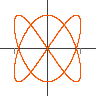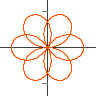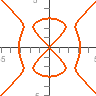Controls Java Version

Plots implicit and algebraic curves like `x^2-y^3`. Type the equation of the curve into box at the bottom or select one from the list on the right. You can click and drag the mouse to shift the screen, and use a mouse wheel to zoom, arrow keys also shift and +, -, PageUp, PageDown zoom. Right clicking brings up a menu with other display options.

The algorithm works by evaluating the equation at every point on the screen, if there is a sign change then a point is plotted. Speed is achieved by using the fasteval Jep extension, this is an evaluator optimized for evaluation over the reals, which is nearly ten times faster than the standard Jep evaluator. You can compare speeds by unticking the Fast method option in the right click menu.

Further speed up for polynomial equations is obtained using a convexity test. First the expression is converted into a polynomial and the coefficents of each term extracted. This is done using the polynomials Jep extension. Then it is converted to a Benstein polynomial which have a simple test for whether the curve may have a zero. (See slides, paper1, paper2) The plotting region is broken up into a number of blocks and this test is caried out on each block to see if the curve needs drawing inside the block.

Jep is a mathematical equation parsing and evaluation library written in java. It features fast evaluation, a configurable architecture allowing custom syntax, support for BigDecimal, complex numbers and vectors It is developed by Singular Systems

GWT-Jep is a port of Jep to run in javascript and has all the features of Jep apart from a small set of changes necessary for javascript compatibility. It is compiled from Java using the Google Web Toolkit. It is available as a seperate package from SingSurf.

Example equations:

• `1+2*3`
• `cos(pi/4)^2`
• `x=5`
• `x^2`

## Jep plotting appsGraphs
`y=a x^2+b x+c`
JavaScript Version
Java VersionParametric
`x=cos(t), y=sin(t)`
JavaScript Version
Java VersionPolar
`r = cos(3 th/2)`
JavaScript Version
Java VersionComplex
`(z+a)/(z+b)`
JavaScript VersionImplicit
`x^2+y^2=1`
JavaScript Version
Java Version